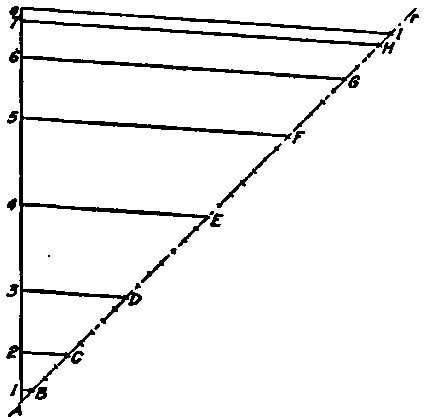For the purpose of illustrating these principles, suppose it is required to design a plate cam, Fig. 90, such that the follower rises from point A to e, with harmonic motion, while the cam rotates through 120°; it is then to remain at rest during the cam movement of 60°; it is then to fall to its original position with a uniformly accelerated and retarded motion, while the cam turns through 150°; it is then to remain at rest while the cam rotates through the remaining 30°, when the same cycle of movement is to be repeated as long as desired. This is briefly expressed as follows:Fig. 89. Method of Dividing Line of Travel.

Harmonic rise............................120°

Rest.................................... 60°

Uniformly accelerated and retarded fall......150°

Rest....................................30°

### 1. Follower Positions

The length of travel Ae being known, it is divided for purposes of the rise, by the principles of Fig. 88, into distances giving harmonic motion of the follower; and for purposes of the fall, by the principles of Fig. 89, into distances giving uniformly accelerated and retarded motion. Eight spaces are chosen, so that the process of making these divisions is an exact repetition of the method in Figs 88 and 89.Fig. 90. Diagram of Complex Motion Plate Cam.

### 2. Cam Radii

The diameter of the base circle being chosen, and, for the purpose of this problem, the center being assumed in line with the path of the follower, draw the original radius CA, then the radii CX, CZ, and CW, limiting the arcs of rise, rest, and fall respectively. As 8 divisions of the follower path have been chosen, the arcs of rise and rest must each be divided into 8 equal divisions. It should be observed that while the divisions are equal throughout each arc, the arc of fall being different from the arc of rise, the divisions of the arc of fall are not equal to the divisions of the arc of rise, measured on the base circle.

### 3. Follower Rotation

The rotation of the follower about the cam, for the period of rise, is accomplished by striking arcs through the points 1,2,3,4,5,6,7, and e, making the intersections R1,R2,R3, R4, R5, R6, R7,and R8, with the cam radii. The rotation of the follower about the cam, for the period of fall, is accomplished by striking arcs through points 1', 2', 3', 4', 5', 6', 7', and e, making the intersections F1,F2, F3, F4,F5, F6, F7,and F8, with the cam radii. These points of intersection represent the centers of the follower roll in its rotated positions, and from these centers should be struck arcs with a radius equal to the radius of the follower roll.

### 4. Tangent Line

A smooth curve is now drawn tangent to these small arcs, thus forming the outline of the cam.

### 5. Testing

The cam may be tested by the tracing-cloth method, precisely as in the cases already developed.

### 6. Pressure Lines

The pressure lines are found in exactly the same manner as in Fig. 81, and can be rotated back to the points in the path of the follower, in order to conveniently study the change in direction of the pressure lines as the follower moves along its path.

It should be noted that in Fig. 90 the same method of procedure is followed as in Figs. 79 to 86, except that the determined points in the path of the follower do not make equal divisions of the path, as in the case of uniform motion.